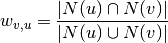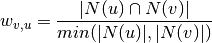NetworkX

#### Previous topic

collaboration_weighted_projected_graph

#### Next topic

generic_weighted_projected_graph

# overlap_weighted_projected_graph¶

overlap_weighted_projected_graph(B, nodes, jaccard=True)[source]

Overlap weighted projection of B onto one of its node sets.

The overlap weighted projection is the projection of the bipartite network B onto the specified nodes with weights representing the Jaccard index between the neighborhoods of the two nodes in the original bipartite network [R124]:or if the parameter ‘jaccard’ is False, the fraction of common neighbors by minimum of both nodes degree in the original bipartite graph [R124]:The nodes retain their attributes and are connected in the resulting graph if have an edge to a common node in the original bipartite graph.

Parameters : B : NetworkX graph The input graph should be bipartite. nodes : list or iterable Nodes to project onto (the “bottom” nodes). jaccard: Bool (default=True) : Graph : NetworkX graph A graph that is the projection onto the given nodes.

Notes

No attempt is made to verify that the input graph B is bipartite. The graph and node properties are (shallow) copied to the projected graph.

References

 [R124] (1, 2, 3) Borgatti, S.P. and Halgin, D. In press. Analyzing Affiliation Networks. In Carrington, P. and Scott, J. (eds) The Sage Handbook of Social Network Analysis. Sage Publications.

Examples

>>> from networkx.algorithms import bipartite
>>> B = nx.path_graph(5)
>>> G = bipartite.overlap_weighted_projected_graph(B, [0, 2, 4])
>>> print(G.nodes())
[0, 2, 4]
>>> print(G.edges(data=True))
[(0, 2, {'weight': 0.5}), (2, 4, {'weight': 0.5})]
>>> G = bipartite.overlap_weighted_projected_graph(B, [0, 2, 4], jaccard=False)
>>> print(G.edges(data=True))
[(0, 2, {'weight': 1.0}), (2, 4, {'weight': 1.0})]